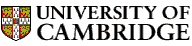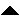Computer LaboratoryComputer Science Syllabus - Numerical Analysis INumerical Analysis INext: Operating Systems II Up: Michaelmas Term 2005: Part Previous: Mathematical Methods for Computer   Contents

## Numerical Analysis I

Lecturer: Dr M.R. O'Donohoe

No. of lectures: 8

This course is a prerequisite for Numerical Analysis II, Digital Signal Processing (Part II).

Aims

The aims of this course are to provide introductions to floating-point arithmetic, numerical analysis and numerical software. Current implementations of floating-point arithmetic will be described. The basic principles of good numerical techniques will be illustrated by examples, but it will be shown that the design of a numerical algorithm is not necessarily straightforward, even for simple problems. The emphasis of the course will be on principles and practicalities rather than mathematical analysis.

Lectures

• Floating-point arithmetic. General description; the numerical analyst's view; overflow and underflow. [0.6 lectures]

• Errors in numerical methods. Machine epsilon; error analysis; solving quadratics; convergence; error testing; rounding error; norms. [1.8 lectures]

• Condition and stability. Condition of a problem; stability of an algorithm. [0.6 lectures]

• Order of convergence; computational complexity. [0.3 lectures]

• IEEE arithmetic. The IEEE Floating-point standards. [1 lecture]

• Simple numerical methods. Differentiation; finite differences; splines. Linear and non-linear equations. Gaussian elimination; Choleski factorisation; linear least squares; Newton-Raphson iteration. Integration. Quadrature rules; summation of series. [3 lectures]

• Numerical software. Portability; languages; the Brown model; implementation issues for IEEE arithmetic, automatic quadrature, BLAS. [0.7 lectures]

Objectives

At the end of the course students should

• appreciate both the historical significance of numerical computation and its continued relevance to the solution of mathematical problems

• understand the advantages and limitations of IEEE arithmetic

• be able to apply a small number of numerical techniques with an understanding of their underlying principles

• understand the special considerations needed when implementing floating-point algorithms in re-usable software© 2005 University of Cambridge Computer Laboratory Please send any comments to pagemaster@cl.cam.ac.uk Page last updated on 11-Sep-2005 at 15:57 by Christine Northeast